# Genetic Algorithms with Apache® Ignite™

These are very exciting times for Apache Ignite. During this past year that I have been with GridGain, I have seen some significant technology additions to the Open Source project, such as support for SQL-99, Native Persistence, and Machine Learning to name but three. Earlier this year, new Genetic Algorithm (GA) code was donated to the Apache Software Foundation. Since I am not very familiar with this technology, I thought it would be a great opportunity to learn about Genetic Algorithms (GAs) and write-up a blog post.

GAs are often used to find very good solutions to complex problems by simulating biological evolution. Some example applications they could be used for include:

• Vehicle design
• Computer games
• Robotics
• Financial investments
• Logistics routing

Biological terminology is often used with GAs. For example, a Population consists of Chromosomes. A Chromosome is a possible solution to a problem. A Chromosome consists of Genes. Genes can be combined to derive new Chromosomes, and so on. A complete glossary can be found in the Apache Ignite GA documentation.

In order to utilize GAs, there are several main operations termed Fitness Calculation, Crossover and Mutation. A Chromosome contains a Fitness Score, which is used to compare different solutions. The process of combining Genes to produce new Chromosomes is called Crossover. Mutation is where some Genes within Chromosomes are updated to produce new characteristics. Graphically, we can see this in Figure 1.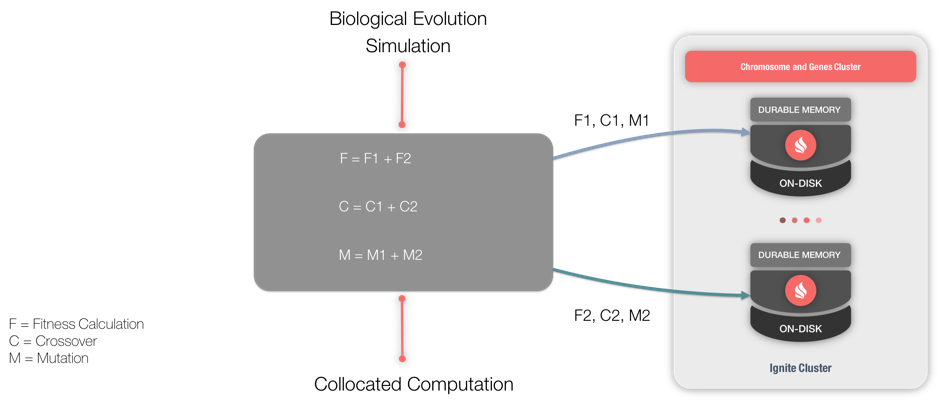Figure 1. GA architecture.

GAs utilize Compute Tasks for their operations and notice the similarity of Figure 1 and the operation of the Apache Ignite Compute Grid, shown in Figure 2.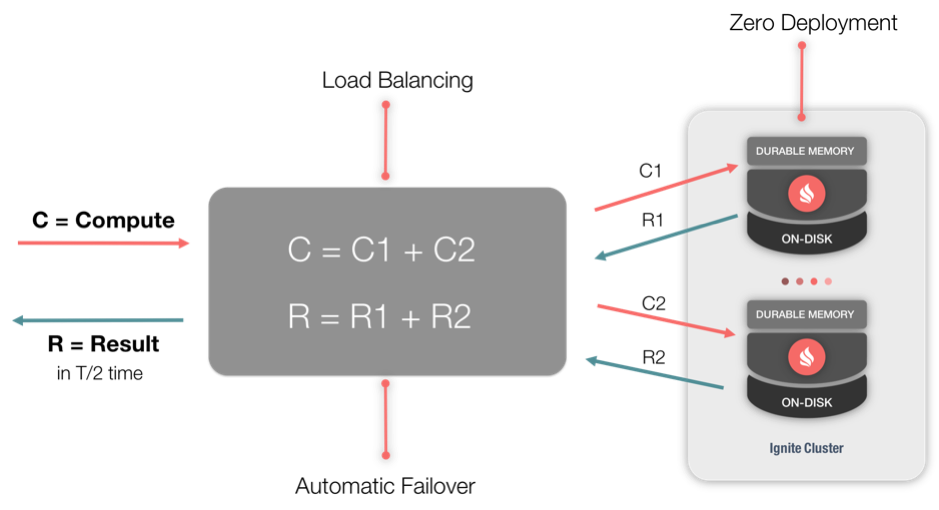Figure 2. Compute Grid.

In Apache Ignite, GAs are part of the larger Machine Learning library.

In Figure 3 (from the Apache Ignite GA documentation), we can see the sequence of operations when using a GA.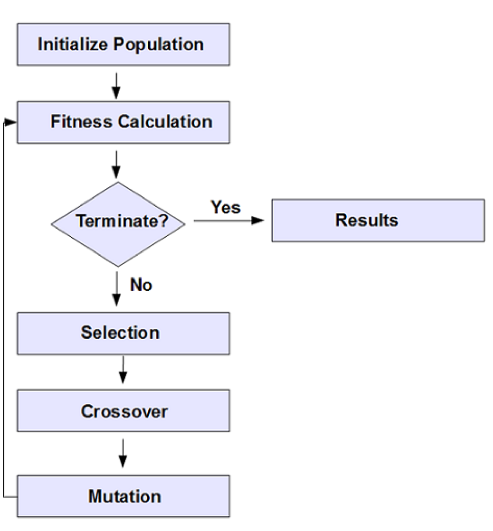Figure 3. GA workflow.

We can see in Figure 3 that an important part of the process is to have a termination step.

Very often in many programming examples, we may find a "Hello World" application. The Apache Ignite GA documentation walks through such an application, step-by-step. Let’s look at this and see how a GA works for this example application.

## "Hello World" Example

The goal of the example is to be able to derive the output "HELLO WORLD". Notice that we are using all capital letters in this case.

The following sections will follow the steps shown in Figure 3. We will use small code snippets to focus on some areas. The complete application code is available on GitHub as part of the larger Apache Ignite Machine Learning library.

### Initialization

First, let's perform some initialization.

``````
ignite = Ignition.start("examples/config/example-ignite.xml");
gaConfig = new GAConfiguration();
```
```

In the code above, we customize GA behavior using GAConfiguration().

Next, we define a Gene Pool, as follows:

``````
List<Gene> genes = getGenePool();
```
```

Our target phrase of "HELLO WORLD" consists of letters in the range A to Z and the space character. Therefore, modeling each Gene as a character gives us a Gene Pool of 27. We can initialize this Gene Pool as follows:

``````
private static List<Gene> getGenePool() {
List<Gene> list = new ArrayList();

char[] chars = {'A', 'B', 'C', 'D', 'E', 'F', 'G', 'H',
'I', 'J', 'K', 'L', 'M', 'N', 'O', 'P', 'Q', 'R', 'S',
'T', 'U', 'V', 'W', 'X', 'Y', 'Z', ' '};

for (int i = 0; i < chars.length; i++) {
Gene gene = new Gene(new Character(chars[i]));
}
return list;
}
```
```

Earlier, we mentioned that a Chromosome is an optimal solution and consists of Genes. In our example, this optimal solution is the phrase “HELLO WORLD”. This consists of 11 Genes. Therefore, we can set the Chromosome length to 11, as follows:

``````
gaConfig.setChromosomeLength(11);
```
```

### Fitness Calculation

To compare different solutions and find the optimal one, we need to determine a Fitness Score for each Chromosome. The following code will enable us to do this:

``````
public class HelloWorldFitnessFunction implements IFitnessFunction {
private String targetString = "HELLO WORLD";

public double evaluate(List<Gene> genes) {

double matches = 0;

for (int i = 0; i < genes.size(); i++) {
if (((Character)(genes.get(i).getValue())).equals(targetString.charAt(i))) {
matches = matches + 1;
}
}
return matches;
}
}
```
```

We can see that our target Chromosome is “HELLO WORLD” and the code checks Gene-by-Gene, to determine if it matches our target.

### Termination Condition

In our example, once a Chromosome matches our target of "HELLO WORLD", we have achieved our goal of finding the optimal solution. So, our code can just check for this condition, as follows:

``````
boolean isTerminate = true;

...

if (!(fittestChromosome.getFitnessScore() > 10)) {
isTerminate = false;
}

return isTerminate;
```
```

### Crossover and Mutation

The final step is to evolve the population, and this can be achieved as follows:

``````
Chromosome fittestChromosome = gaGrid.evolve();
```
```

### Running the GA

Finally, we are ready to run the code. Some example output is shown below:

```##########################################################################################
Generation: 255
Fittest is Chromosome Key: Chromosome [fitnessScore=11.0, id=131, genes=[8, 5, 12, 12, 15, 27, 23, 15, 18, 12, 4]]
Chromosome: Chromosome [fitnessScore=11.0, id=131, genes=[8, 5, 12, 12, 15, 27, 23, 15, 18, 12, 4]]
HELLO WORLD
Avg Chromosome Fitness: 5.668
##########################################################################################```

## Web Console

We can also run some GA queries using the Apache Ignite Web Console. To see this in use, we start a cluster node either from the command line or from an IDE. Once the node is up and running, we start the Ignite Web Agent. Figure 4 shows the Web Agent running.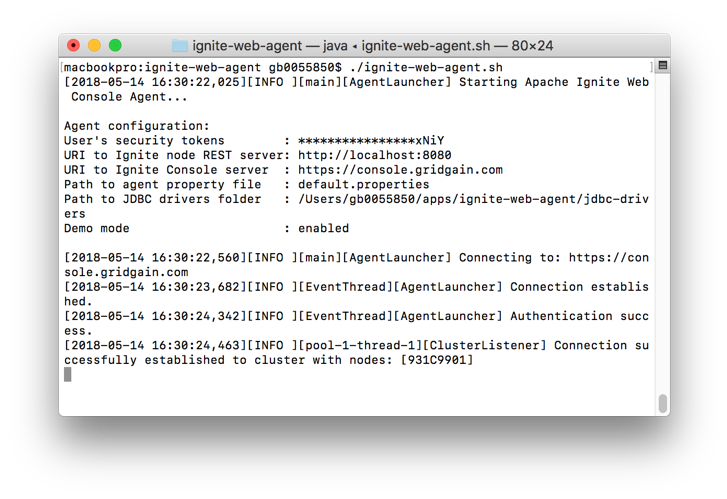Figure 4. Ignite Web Agent.

Next, we use a web browser and connect to http://console.gridgain.com. Once logged-in, we navigate to Queries > Create new notebook. After naming and creating the new notebook, we are presented with an interface where we can run SQL queries, as shown in Figure 5.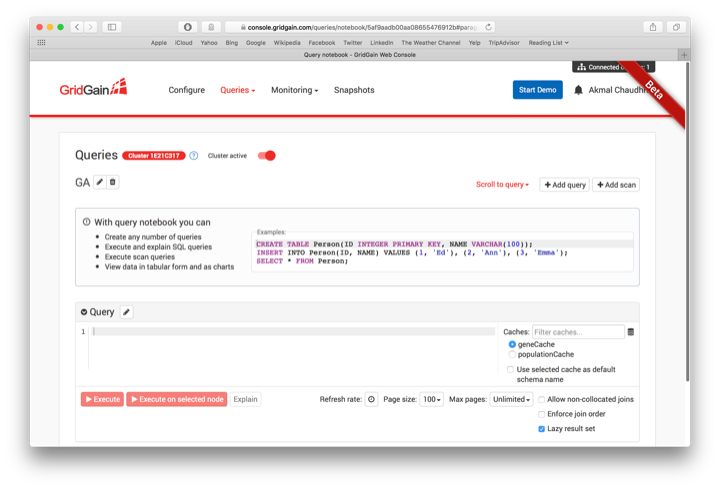Figure 5. Web Console.

From the GA documentation:

GA provides improved knowledge discovery by adding custom SQL functions to pivot genetic optimization results. Columns in a result set are dynamically driven by the Chromosome size for an individual genetic sample.

Several SQL functions are available:

• getSolutionsDesc(): Retrieves optimal solutions in descending order based upon the fitness score.
• getSolutionsAsc(): Retrieves optimal solutions in ascending order based upon the fitness score.
• getSolutionById(key): Retrieves an optimal solution by Chromosome key.

Let's try the following example:

`select * from "geneCache".getSolutionsDesc();`

This produces the output shown in Figure 6.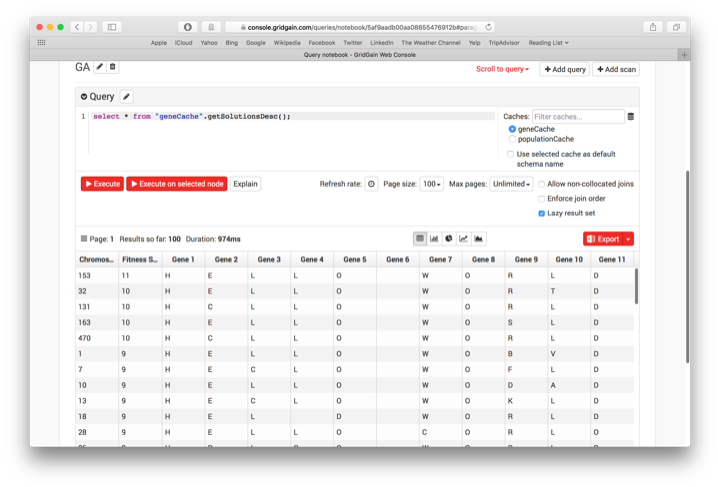Figure 6. SQL Function output.

From the output, we can see that the first solution is the best with a Fitness Score of 11.

## Summary

A GA provides a powerful mechanism for solving some complex problems using a process that simulates biological evolution. Through a simple example, we have seen GAs at work. Further GA code examples are also available through the Apache Ignite Machine Learning library.